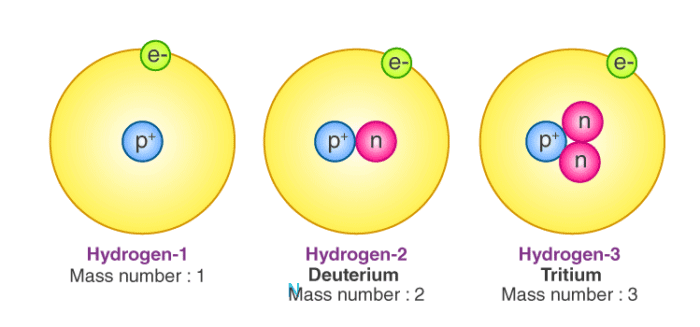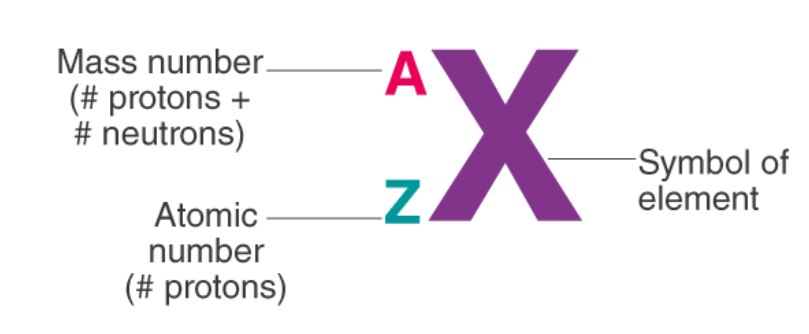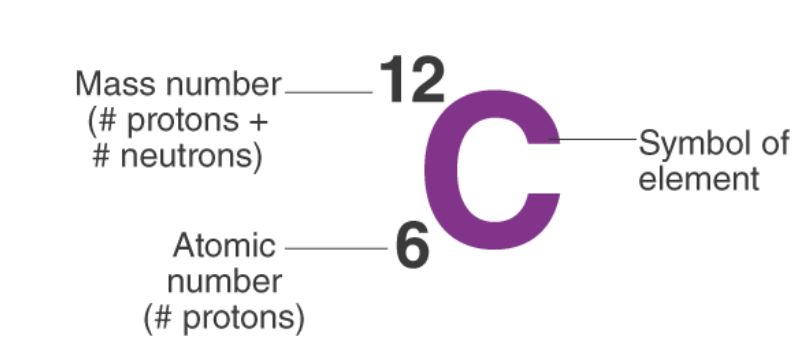Atomic Number and Mass Number

# Atomic Number and Mass Number - Science Class 9

Atomic number and mass number are always whole numbers because they are obtained by counting whole objects (protons, neutrons, and electrons). The sum of the mass number and the atomic number for an atom (A-Z) corresponds to the total number of subatomic particles present in the atom. The mass number reports the mass of the atom’s nucleus in atomic mass units (amu).
The modern periodic table is arranged in such a way that all the elements have an increasing atomic number, and subsequently, increasing mass number. But do you know what mass number, or even what atomic number is?
Well, as you know, an atom consists of electrons, protons and neutrons. The number of electrons in the outermost shell gives us the valency of the atom. Similarly, the number of protons and neutrons are associated with the atomic number and mass number of the atom.

Let’s look at the difference between the two terms.

What is Atomic Number?

• The total number of protons in the nucleus of an atom gives us the atomic number of that atom.
• It is represented with the letter ‘Z.’
• All the atoms of a particular element have the same number of protons, and hence the same atomic number.
• Atoms of different elements have different atomic numbers.
• For example, all carbon atoms have the atomic number of 6, whereas all atoms of Oxygen have 8 protons in their nucleus.

What is Mass Number?

• The number of protons and neutrons combined to give us the mass number of an atom.
• It is represented using the letter ‘A.’
• As both protons and neutrons are present in the nucleus of an atom, they are together called nucleons.
• For example, an atom of carbon has 6 protons and 6 neutrons. Thus, its mass number is 12.
• While the number of protons remains the same in all atoms of an element, the number of neutrons can vary. Thus, atoms of the same element can have different mass numbers, and these are called isotopes.
• The weight of an electron is almost negligible. Thus, the atomic mass of an atom is almost the same as its mass number.

History of Atomic Number

We take the existence of atoms for granted – yet for centuries no one believed in them. Around 2500 years ago a Greek philosopher called Leucippus and his pupil Demokritos put forward the idea that the universe is made up of tiny indivisible particles, which they called atoms. Unfortunately the great Greek philosopher Aristotle did not agree with them. As Aristotle views were accepted throughout Europe for almost 2000 years the idea of atoms was shelved for centuries to come.
Atoms are the building blocks of matter. They combine in numerous patterns and form different substances. All atoms except the common form of hydrogen contain protons, neutrons and electrons. The atomic number of an element is equal to the number of protons in its nucleus. In a neutral atom, the number of protons equals the number of electrons in shells that is the energy level around the nucleus.

Examples of Atomic Number

The atomic number of an atom is equal to the number of protons in the nucleus of an atom or the number of electrons in an electrically neutral atom.

Atomic number = Number of protons
For example, in a sodium atom, there are 11 electrons and 11 protons. Thus the atomic number of Na atom = number of electrons = number of protons = 11.

Atomic Number Orbital Energy Levels

When an electron is at a specific energy level, it is more likely to be found in certain portions of that level than others. Orbitals are the name for these sections. Sublevels are made up of orbitals with the same energy. A maximum of two electrons can be found in each orbital. The most common way of showing the arrangement of electrons in an atom is to draw diagrams like those shown in the diagram.To write down the numbers of electrons in each energy level. The atomic number of an element tells us how many electrons there are in the atoms. For example, the atomic number of carbon is 6 giving us six electrons as 2,4. So an atom with the atomic number 12 has an electronic structure 2, 8, 2, with two electrons in the inner energy level, then eight in the next energy level and two in the outer highest energy level. The simplest way to understand these arrangements is to look at lots of examples of them.

Difference between Valency, A and Z

When we want to find out the valency, we look at electrons only in the outermost shell of the atom. But when we want to know the atomic number or the mass number, we look at the total number of protons and neutrons.
1. Notation of Atom
To write the notation of an atom, we need to know the symbol of the element, the atomic number and the mass number. The mass number of the atom goes above the symbol and the atomic number is written as a subscript.So, the notation of Carbon is:2. Calculating Number of Neutrons
If we know the number of protons and the mass number of an element, we can also calculate the number of neutrons simply by subtracting its atomic number from its mass number.
Number of neutrons = A - Z

### Solved Example

Question: An atom has an atomic number of 9 and a mass number of 19.

1. Determine the number of protons present
2. Determine the number of neutrons present
3. Determine the number of electrons present

Solution:

1. There are 9 protons because the atomic number is always equal to the number of protons present.
2. There are 10 neutrons because the number of neutrons is always obtained by subtracting the atomic number from the mass number.
(protons + neutrons) – protons = neutrons
3. There are 9 electrons because the number of protons and the number of electrons are always the same in an atom.
The document Atomic Number and Mass Number | Science Class 9 is a part of the Class 9 Course Science Class 9.
All you need of Class 9 at this link: Class 9

## Science Class 9

66 videos|352 docs|97 tests

## FAQs on Atomic Number and Mass Number - Science Class 9

 1. What is the atomic number and mass number of an element?Ans. The atomic number of an element represents the number of protons in the nucleus of an atom. It determines the element's identity and is denoted by the symbol "Z" in the periodic table. On the other hand, the mass number of an element is the sum of protons and neutrons in the nucleus. It is denoted by the symbol "A" and gives an idea about the mass of an atom.
 2. How are atomic number and mass number related?Ans. The atomic number and mass number are related as follows: the atomic number (Z) represents the number of protons, while the mass number (A) represents the sum of protons and neutrons in the nucleus. Therefore, the number of neutrons in an atom can be calculated by subtracting the atomic number from the mass number (N = A - Z).
 3. Can the atomic number and mass number of an element be the same?Ans. No, the atomic number and mass number of an element cannot be the same. The atomic number (Z) is always a whole number and represents the number of protons, which uniquely identifies an element. On the other hand, the mass number (A) is a whole number representing the sum of protons and neutrons, which may vary for different isotopes of the same element.
 4. How can atomic number and mass number be used to determine the number of electrons in an atom?Ans. In a neutral atom, the number of electrons is equal to the number of protons, which is represented by the atomic number (Z). Therefore, to determine the number of electrons in an atom, one can simply look at the atomic number of the element. For example, carbon (C) has an atomic number of 6, so it contains 6 electrons.
 5. Is the atomic number or mass number more important in determining an element's properties?Ans. Both the atomic number and mass number play significant roles in determining an element's properties. The atomic number determines the element's identity and its position in the periodic table, which directly influences its chemical properties. The mass number, on the other hand, affects the atomic mass and isotopic abundance, which can have implications for nuclear stability and physical properties. Therefore, both numbers are essential in understanding an element's characteristics.

## Science Class 9

66 videos|352 docs|97 testsExplore Courses for Class 9 examSignup to see your scores go up within 7 days! Learn & Practice with 1000+ FREE Notes, Videos & Tests.
10M+ students study on EduRev
Track your progress, build streaks, highlight & save important lessons and more!
Related Searches

,

,

,

,

,

,

,

,

,

,

,

,

,

,

,

,

,

,

,

,

,

;